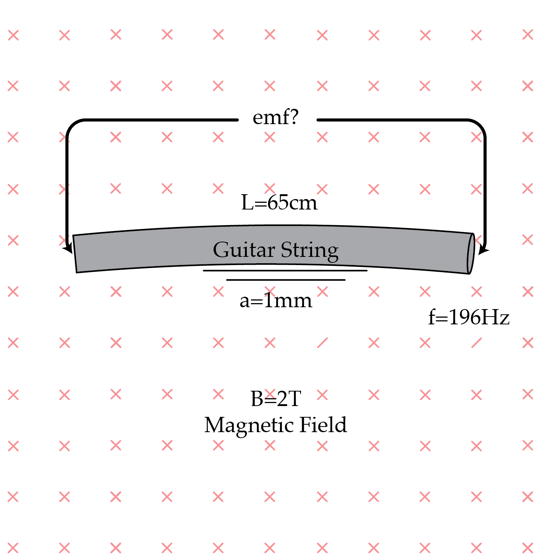# Emf on the G string

The fundamental frequency of the G string of a guitar is $f= 196 Hz$. The fundamental vibrational mode of the string is described by the standing wave $y(t,x)= a \cos(2 \pi f t) \sin(\frac{\pi x}{L})$ where $L=65 cm$ is the length of the string and $a=1mm$ is the amplitude of the oscillations. What would be the peak emf in Volts induced across the ends of the string if the guitar is played in a $B= 2T$ magnetic field?

Assume that the magnetic field is perpendicular to the plane in which the string vibrates.×